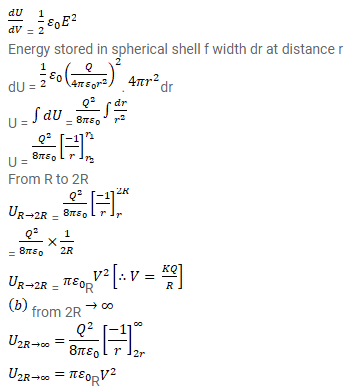# A metal sphere of radius R is charged to a potential V.Question:

A metal sphere of radius $\mathrm{R}$ is charged to a potential $\mathrm{V}$.

(a) Find the electrostatic energy stored in the electric field within a concentric sphere of radius $2 \mathrm{R}$.

(b) Show that the electrostatic field energy stored outside the sphere of radius $2 \mathrm{R}$ equals that stored within it.

Solution:hence proved